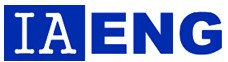random

random
recent
recent

# IAENG International Journal of Applied Mathematics

IAENG International Journal of Applied Mathematics### Objectives and Scope

IAENG International Journal of Applied Mathematics is published with both online and print versions. The journal covers the frontier issues in the applied mathematics and their applications in business, industry, science and other subjects. Applied Mathematics is a branch of mathematics that concerns itself with the connections between mathematics and other domains with the applications of the mathematical knowledge. A broad spectrum of applied mathematics is covered by the journal. The subjects include differential equations (ODEs and PDEs), numerical analysis, scientific computing, approximation theory and representation theory, matrix theory, mathematical physics, mathematical methods of engineering, optimization, operations research, linear and nonlinear programming, continuous modelling control theory, mathematical biology, bioinformatics, information theory, game theory, probability, mathematical economics, financial mathematics, actuarial science, cryptography, graph theory, statistics, theoretical computer science, stochastic systems theory, neuroscience, mechanics of solids, materials science and fluids etc.

Printed copies of the journal are distributed to accredited universities and government libraries. All the papers in the journal are also available freely with online full-text content and permanent worldwide web link. The abstracts will be indexed and available at major academic databases (like for example Scopus and EI Compendex).

• Frequency: 4 issues per year
• ISSN: 1992-9986 (online version); 1992-9978 (print version)
• Subject Category: Applied Mathematics
• Coming Special Issues of the IAENG International Journals
• Web: http://www.iaeng.org/IJAM/

IAENG International Journal of Applied Mathematics welcomes author submission of papers concerning any branch of the applied mathematics and their applications in business, industry and other subjects. The subjects include differential equations (ODEs and PDEs), numerical analysis, scientific computing, approximation theory and representation theory, matrix theory, mathematical physics, mathematical methods of engineering, optimization, operations research, linear and nonlinear programming, continuous modelling control theory, mathematical biology, bioinformatics, information theory, game theory, probability, mathematical economics, financial mathematics, actuarial science, cryptography, graph theory, statistics, theoretical computer science, stochastic systems theory, neuroscience, mechanics of solids, materials science and fluids etc.

All submitted papers are to be peer-reviewed for ensuring their qualities.

If you like the content of our siteweb, we hope to stay in touch, just enter your e-mail to subscribe to the blog post to get the new and you can send a message by clicking the button next to ...